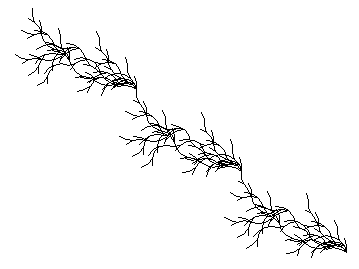# Welcome to the QChartist blogQChartist Community – Your way to financial freedom

## February 21, 2023

### L-Systems

Filed under: Fractals — admin @ 2:37 pm

Lindenmayer L-Systems Rules
Creating turtle fractal art with L-Systems just for the fun

These fractals are created with my free L-System app that you can download here:
First install Python 3.8 for Windows :
http://www.qchartist.net/files/programming/fractals/python-3.8.0.exe
Then extract this zip file in a folder inside your Python38-32 folder :
http://www.qchartist.net/files/programming/fractals/lsystem-master_RQ_Py.zip
Enjoy!

https://www.deviantart.com/jul-zen

Hive
Axiom: F
Production 1: F
Rule 1: +F++DF++D[F]D+
Production 2: D
Rule 2: D
Iterations: 7
Angle: 30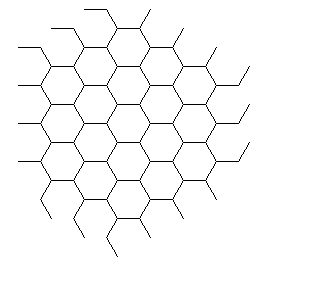von Koch – acute snowflake
axiom=”F+(120)F+(120)F”
rules={“F”: “F-F++F-F”}
iters=4
angle=75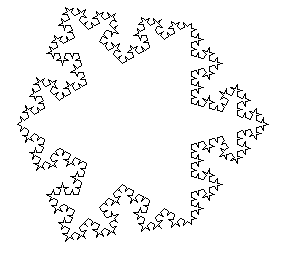Cesaro curve #1
axiom=”F+(90)F+(90)F+(90)F”
rules={“F”: “F+F–F+F”}
iters=5
angle=85.3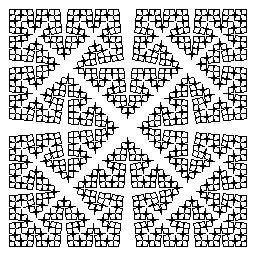Mango Leaf
axiom=”Y—Y”
rules={“X”: “F-FF-F–[–X]F-FF-F–F-FF-F–“, “Y”: “f-F+X+F-fY”}
iters=10
angle=60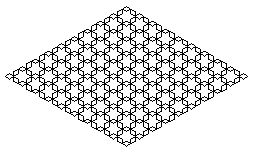Weed, P. Bourke
axiom=”F”
rules={“F”: “FF-[XY]+[XY]”,”X”:”+cFY”,”Y”:”-dFX”,”c”:””,”d”:””}
iters=6
angle=22.5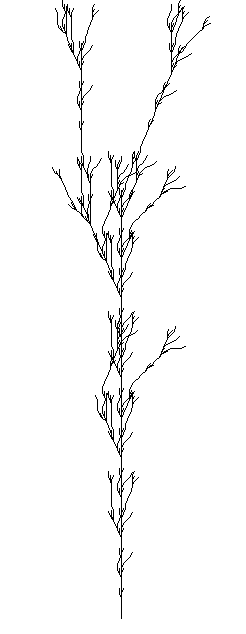poetasters weed, after, P. Bourke
axiom=”F”
rules={“F”: “F-[XY]+[XY]F+[XY]-[XY]”,”X”:”+dFY”,”Y”:”-cFX”,”c”:””,”d”:””}
iters=6
angle=22.5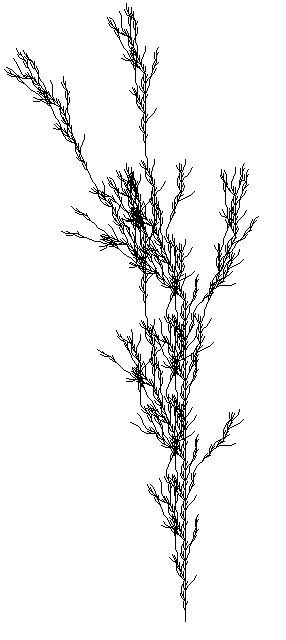Unlikely bush (after Bourke)
axiom=”F”
rules={“F”: “eF[+cFF][-FF]cF[-F]d[+F]F”,”c”:””,”d”:””,”e”:””}
iters=3
angle=330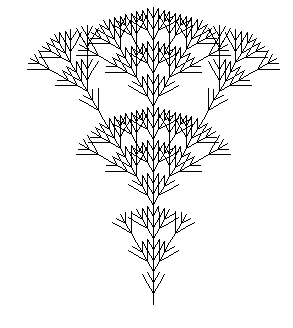von Koch – islands and lakes
axiom=”F+F+F+F”
rules={“F”: “F+f-FF+F+FF+Ff+FF-f+FF-F-FF-Ff-FFF”, “f”: “ffffff”}
iters=2
angle=90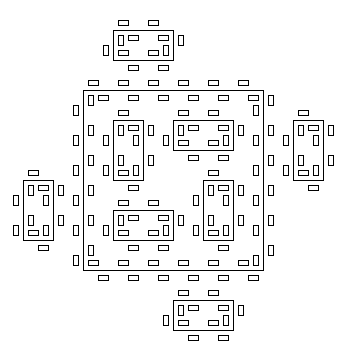2D bush #9
axiom=”G”
rules={“G”: “GfX[+G][-G]”, “X”: “X[-FFF][+FFF]FX”}
iters=5
angle=25.71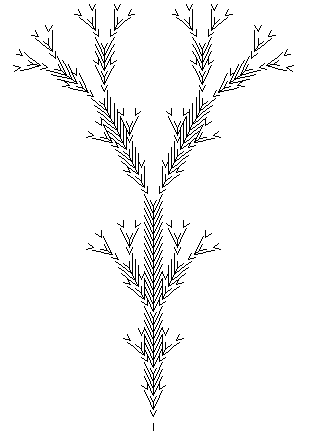Snake Kolam
axiom=”F+XF+F+XF”
rules={“X”: “XF-F-F+XF+F+XF-F-F+X”}
iters=4
angle=90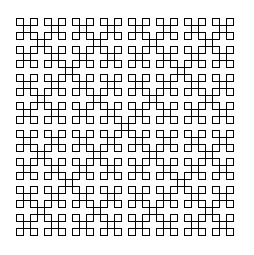peano_gosper_curve_1
axiom=”FX”
rules={“X”: “X+YF++YF-FX–FXFX-YF+”, “Y”: “-FX+YFYF++YF+FX–FX-Y”}
iters=4
angle=60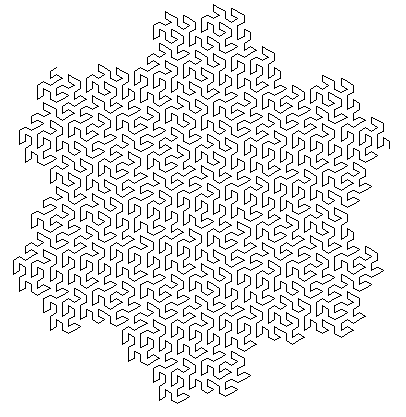segments 1
axiom=”FB”
rules={“A”: “FBFA+HFA+FB-FA”, “B”: “FB+FA-FB-JFBFA”, “H”: “-“, “J”: “+”, “F”: “”}
iters=5
angle=90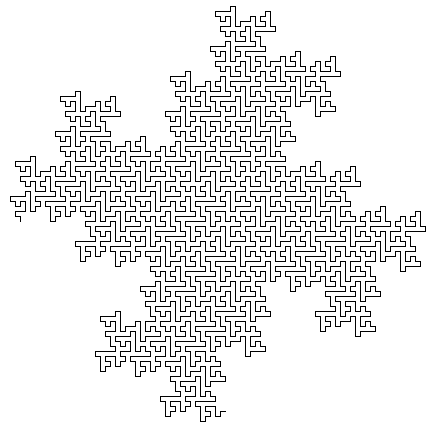Tree
axiom=”X”
rules={“F”: “FF”, “X”: “F-[[X]+X]+F[+FX]-X”}
iters=6
angle=22.5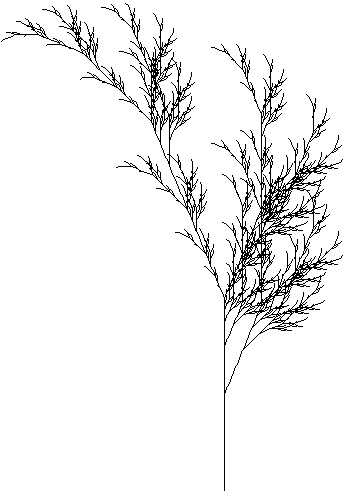Wheel
axiom=”WWWWWWWWWWWWWWWWWWWWWWWWWWWWWWWWWWWWWWWWWWWWWW”
rules={“W”: “[X]+”, “X”: “[+++++++++++++Y[X]]————-Y[X]”, “Y”: “YFYF”}
iters=8
angle=5Tree 2
axiom=”FFFFFFX”
rules={“F”: “F”, “X”: “FFF-[-F+F[Y]-[X]]+[+F+F[X]-[X]]”, “Y”: “FF-[-F+F]+[+F+FY]”}
iters=6
angle=15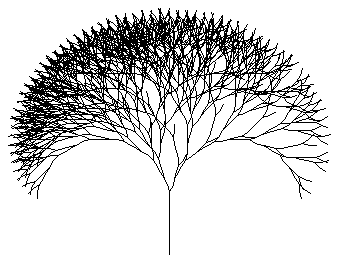P. Bourke after Saupe
axiom=”VZFFF”
rules={“V”: “[+++W][—W]YV”,”W”:”+X[-W]Z”,”X”:”-W[+X]Z”,”Y”:”YZ”,”Z”:”[-FcFF][+FdFF]F”,”c”:””,”d”:””}
iters=8
angle=20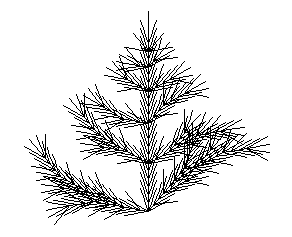poetasters succulent 1
axiom=”A”
rules={“A”: “[FL]gAhg[FLA]”,”F”:”cSF”,”S”:”dFL”,”L”:”c[F+F+F]fe[F-F-F]”,”c”:””,”d”:””,”e”:””,”g”:””,”h”:””}
iters=7
angle=17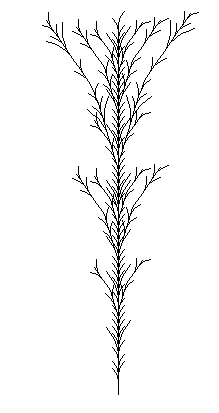Pean-c flower after Gary Teachout
axiom=”FXhFXiFX”
rules={“F”: “”,”X”:”[FX-FY][-cFX-FY-FX][ZZ]-dFY-FX+FY+FX”,”Y”:”FY”,”Z”:”-cFX-FY-FX”,”c”:””,”d”:””,”h”:””,”i”:””}
iters=3
angle=340